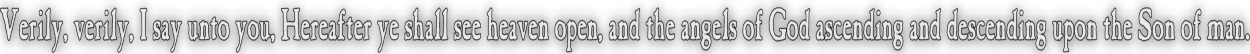None:
Polyps:
Strongs:CreationismThe Trumpets To Wormwood Quickly, a recap on the trumpet devices leading to the brackish water of "Death" also called "Wormwood" in the book of revelation. Starting with a single octal as a reference, origin or "sun octal" we have eight possible C7 groups that preserve the subgroups under multiplication. so for ([a,b,c],[a,d,e],[a,f,g],[b,d,f],[b,e,g],[c,e,f,[c,d,g]) we may have any of the eight seven cycles (a,b,d,c,f,g,e) (a,b,f,c,d,e,g) (a,c,d,b,g,f,e) (a,c,g,b,d,e,f) (a,b,e,c,g,f,d) (a,b,g,c,e,d,f) (a,c,e,b,f,g,d) (a,c,f,b,e,d,g) Where in their pairs they hold static the subgroups [b,d,f], [c,d,g], [b,e,g] and [c,e,f] respectively. On choosing any three of these possible multiplications, say the 1st, 3rd and 5th, we may construct upon the subgroups various 2nd generation octals from our origin, our reference or "sun" octal. a = (b,d,f) = {c,e,g} b = (c,d,g) = {a,e,f} d = (c,e,f) = {a,b,g} c = (a,f,g) = {b,d,e}* f = (b,e,g) = {a,c,d} g = (a,f,g) = {b,c,d} e = (a,b,c) = {d,f,g}# and a = (c,d,g) = {b,e,f} c = (b,d,f) = {a,e,g} d = (b,e,g) = {a,c,f} b = (a,f,g) = {c,d,e} g = (c,e,f) = {a,b,d} f = (a,d,e) = {b,c,g}\$ e = (a,b,c) = {d,f,g}# and a = (b,e,g) = {c,d,f} b = (c,e,f) = {a,d,g} e = (c,d,g) = {a,b,f} c = (a,f,g) = {b,d,e}* g = (b,d,f) = {a,c,e} f = (a,d,e) = {b,c,g}\$ d = (a,b,c) = {e,f,g} Now, note that each of the three contains one shared row with each of the remaining two: here marked with *, # and \$. We should note these rows correspond to the elements of a subgroup in our reference octal, so we may equate the 2nd generation octals in each case to the product of the two rows in the other two. So the first is c + e = f, the second is e + f = c and the third c + f = e. Now, we introduce our "wormwood" octal, also a valid octal pair, yet containing the missing rows from the three above, so we must find an octal that contains all three rows of the missing product, each octals own "self corresponding row" . We need an octal that contains the rows... f = (b,e,g) = {a,c,d} ! c = (b,d,f) = {a,e,g} ! e = (c,d,g) = {a,b,f} ! Keeping a = 1 for simplicities sake, which is the intersection of the left hand triples - we know that it holds static (c,e,f) when a = 1. Thus by inspection we quickly obtain; (a,c,f,b,e,d,g) which generates the octal a = (c,e,f) = {b,d,g} c = (b,d,f) = {a,e,g} ! f = (b,e,g) = {a,c,d} ! b = (a,d,e) = {c,f,g} e = (c,d,g) = {a,b,f} ! d = (a,f,g) = {b,c,e} g = (a,b,c) = {d,e,f} Which contains the exact same set of rows marked (!), so that we note that the rows from the three octals above are found in this one octal. However: there must be a second multiplication on the octal that contains the same pair of a = (c,e,f). Yet this arrangement does not associate with the two other rows needed. To intents and purposes, the "wormwood" octal is unique on the same reference or "sun octal". We move on to discuss the implications for the octal sea under the devices of satan, the hurting of the oil and wine of this section. Continue To Next Page Return To Section Start'